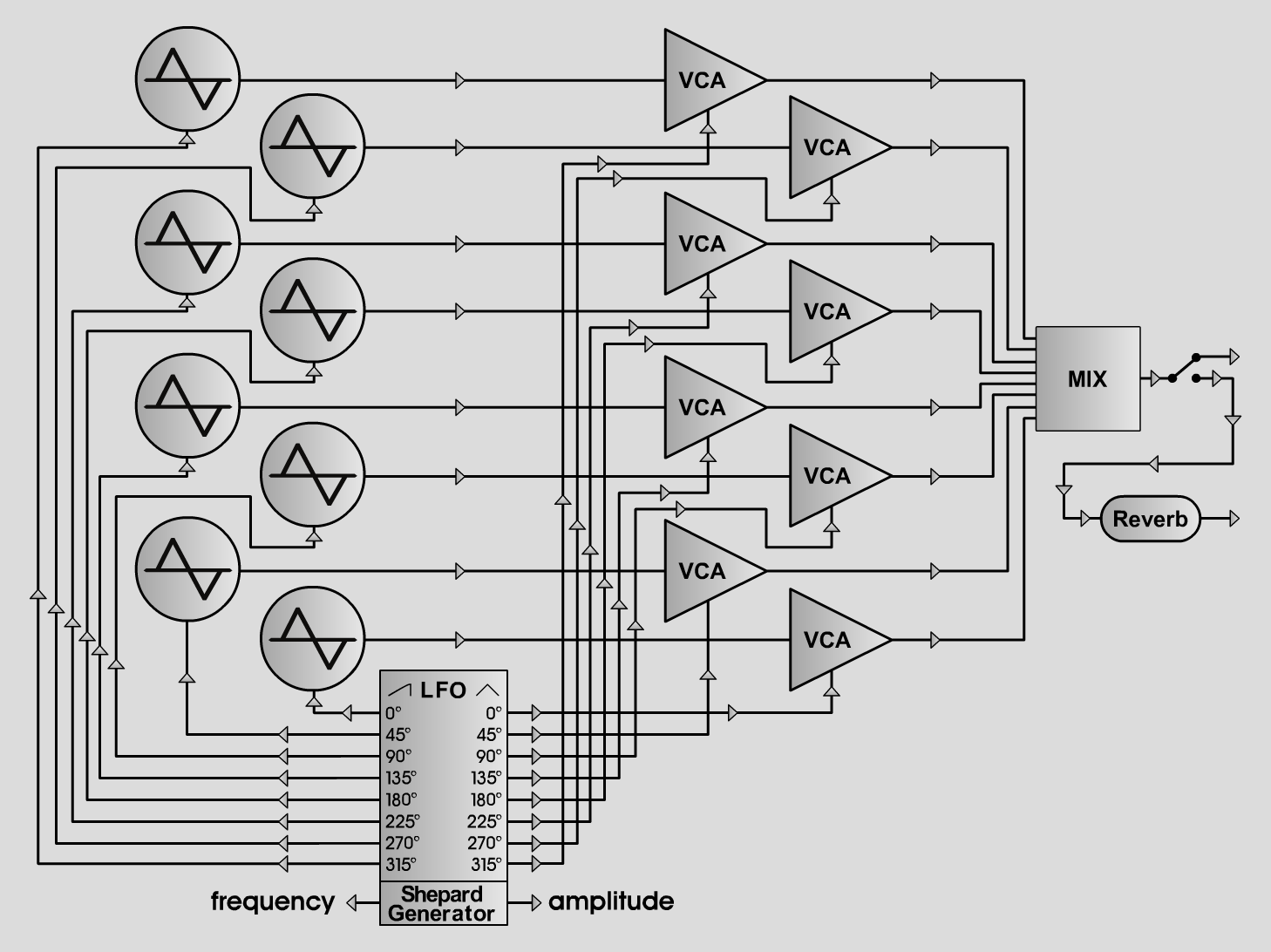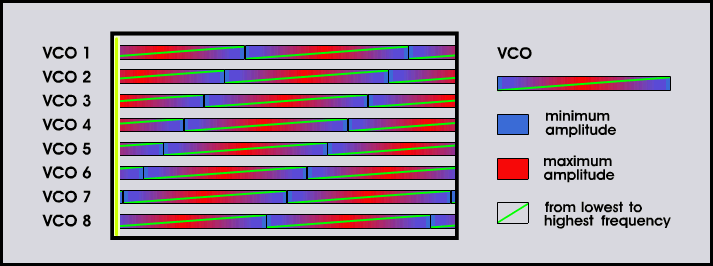Shepard-ToneExperimentalD o e p f e r A - 100 Connections*: Settings: Shepard-high-Tri-Rev: A-191 (S0) <=> A-111/1 (CV1)  A-191 (S45) <=> A-111/2 (CV1)  A-191 (S90) <=> A-111/3 (CV1)  A-191 (S135) <=> A-111/4 (CV1)  A-191 (S180) <=> A-111/5 (CV1)  A-191 (S225) <=> A-111/6 (CV1)  A-191 (S270) <=> A-110/1 (CV1)  A-191 (S315) <=> A-110/2 (CV1)  A-191 (T0) <=> A-135 (CV1)  A-191 (T45) <=> A-135 (CV2)  A-191 (T90) <=> A-135 (CV3)  A-191 (T135) <=> A-135 (CV4)  A-191 (T180) <=> A-132-3/top (CV In)  A-191 (T225) <=> A-132-3/bottom (CV In)  A-191 (T270) <=> A-132/top (CV 1)  A-191 (T315) <=> A-132/bottom (CV 1)  A-111/1 (Tri) <=> A-135 (Audio In 1)  A-111/2 (Tri) <=> A-135 (Audio In 2)  A-111/3 (Tri) <=> A-135 (Audio In 3)  A-111/4 (Tri) <=> A-135 (Audio In 4)  A-111/5 (Tri) <=> A-132-3/top (In)  A-111/6 (Tri) <=> A-132-3/bottom (In)  A-110/1 (Tri) <=> A-132/top (Sig. In)  A-110/2 (Tri) <=> A-132/bottom (Sig. In)  A-132-3/top (Out) <=> A-138/2exp (Input 1)  A-132-3/bottom (Out) <=> A-138/2exp (Input 2)  A-132/top (Sig Out) <=> A-138/2exp (Input 3)  A-132/bottom (Sig Out) <=> A-138/2exp (Input 4)  A-138/2exp (Output) <=> A-138/1exp (Input 2)  A-135 (Audio Out) <=> A-138/1exp (Input 1)  A-138/1exp (Output) <=> A-182 (A)  A-182 (B) <=> A-131 (Audio In 1)  A-182 (C) <=> A-199 (Audio In)  A-199 (Mix Out) <=> A-182 (D)  A-131 (Audio Out) <=> Amp A-191 (Mode: rising sawtooth)  A-111/1-6 (Octave = 0, Tune = 5, Fine = 7, PW = 5)  A-110/1-2 (Range = 1, Tune = 9, PW = 5)  A-135 (Audio In 1-4 = 10, Gain 1-4 = 0, ext. CV 1-4 = 10)  A-135 (Audio In 1-4 = 10, Gain 1-4 = 0, ext. CV 1-4 = 10)  A-132-3/top+bottom (CV = 10, Gain = 0, lin.)  A-138/1exp (In 1 = 10, In 2 = 10, In 3 = , In 4 = , Out = 7)  A-138/2exp (In 1 = 7.5, In 2 = 7.5, In 3 = 6.5, In 4 = 6.5, Out = 10)  A-199 (Level = 8, Feedback = 0, Emphasis = 0, Mix = 6/4)  A-182 (A = 1, B = 2, C = 1, D = 2)  A-131 (Gain = 10, Audio In 1 = 6, Audio Out = 8) Shepard-mid-Pulse-Rev: A-111/1-6 (Pulse)  A-110/1-2 (Pulse) A-111/1-6 (Octave = -1, Tune = 5, Fine = 7, PW = 5)  A-110/1-2 (Range = 0, Tune = 9, PW = 5) Shepard-mid-Saw-Rev: A-111/1-6 (Saw)  A-110/1-2 (Saw) A-111/1-6 (Octave = -1, Tune = 5, Fine = 7, PW = 5)  A-110/1-2 (Range = 0, Tune = 9, PW = 5) Shepard-falling-mid-Pulse-Rev: A-111/1-6 (Pulse)  A-110/1-2 (Pulse) A-111/1-6 (Octave = -1, Tune = 5, Fine = 7, PW = 5)  A-110/1-2 (Range = 0, Tune = 9, PW = 5)  A-191 (Mode: falling sawtooth) Without Reverb: A-182 (A = 1, B = 1, C = off, D = off) Connections Shepard Generator => Pulse Width: Settings: Shepard-PW-low: A-111/1-6 (Pulse)  A-110/1-2 (Pulse)  A-191 (S0 - S225) <=> A-111/1-6 (PCV)  A-191 (S270 - S315) <=> A-110/1-2 (PW CV2) A-111/1-6 (Octave = -1, Tune = 5, Fine = 7, PW = 5, PCV = 5)  A-110/1-2 (Range = 0, Tune = 9, PW = 5, PW CV2 = 5) Shepard-PW-mid: A-111/1-6 (Octave = 0, Tune = 5, Fine = 7, PW = 5, PCV = 5)  A-110/1-2 (Range = 1, Tune = 9, PW = 5, PW CV2 = 5) Shepard-PW-high: A-111/1-6 (Octave = 1, Tune = 5, Fine = 7, PW = 5, PCV = 5)  A-110/1-2 (Range = 2, Tune = 9, PW = 5, PW CV2 = 5) Shepard-PW-mix: A-111/1-6 (Tune = 5, Fine = 7, PW = 5, PCV = 5)  A-111 (Octave: 1 = -1, 2 = +1, 3 = -1, 4 = +1, 5 = -1, 6 = +1)  A-110/1-2 (Range = 0, Tune = 9, PW = 5, PW CV2 = 5)  A-110 (Range: 1 = 0, 2 = +2) * realized as Shepard-Risset Glissando Josef MuellerSound samples Shepard-Piece   (Theme from Bach BWV 244 Nr. 8)       2. sound: Bell                  3. sound: Marsians                  4. sound: Grandfather's Clock Shepard-high-Tri-Rev Shepard-mid-Pulse-Rev Shepard-mid-Saw-Rev Shepard-falling-mid-Pulse-Rev Sound samples without A-199 (reverb): Shepard-high-Tri Shepard-mid-Pulse Shepard-mid-Saw Shepard-falling-mid-Pulse Shepard-PW-low Shepard-PW-mid Shepard-PW-high Shepard-PW-mix